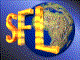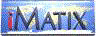| iMatix home page | << | < | > | >>SFLVersion 2.11

```#include "sflnode.h"
void *
void *left,
void *node,
void *right)
```

#### Synopsis

Links the node into a linked list. This is a general-purpose function that can be used to attach and remove nodes anywhere in a list. Sets the global variable 'node_unsafe' while the list is being changed. Returns the address of node.

#### Source Code - (sflnode.c)

```{
NODE *swap;

node_unsafe = TRUE;
swap = ((NODE *) left)-> next;      /*  Exchange left pointers           */
((NODE *) left)-> next = ((NODE *) node)-> next;
((NODE *) node)-> next = swap;

swap = ((NODE *) right)-> prev;     /*  Exchange right pointers          */
((NODE *) right)-> prev = ((NODE *) node)-> prev;
((NODE *) node)-> prev = swap;
node_unsafe = FALSE;
return (node);
}
```

 | << | < | > | >>Copyright © 1996-2000 iMatix Corporation